title: A Fair Price for the Republic Gunship? A Bayesian Analysis of Ultimate Collector Series Prices in Python with PyMC3

## tags: Lego, Python, PyMC3, Bayesian¶

Since my last post on modeling Lego prices, Lego has announced the release of 73509, an Ultimate Collector Series Republic Gunship from the Battle of Geonosis.As with 75296, Darth Vader Meditation Chamber, we can ask whether the $349.99 recommended retail price of this set is fair given its number of pieces, Star Wars theme, and Ultimate Collector Series status. This post will update the model from the previous one to include random effects for subtheme (in Lego collector jargon, the theme of 75309 is "Star Wars" and the subtheme is "Ultimate Collector Series") to answer this question. In addition, we will explore the effect of different themes and subthemes on set prices. First we make some Python imports and do a bit of housekeeping. In : %matplotlib inline  In : import datetime from functools import reduce from warnings import filterwarnings  In : from aesara import shared, tensor as at import arviz as az from matplotlib import MatplotlibDeprecationWarning, pyplot as plt from matplotlib.ticker import StrMethodFormatter import numpy as np import pandas as pd import pymc3 as pm from sklearn.preprocessing import StandardScaler import seaborn as sns  You are running the v4 development version of PyMC3 which currently still lacks key features. You probably want to use the stable v3 instead which you can either install via conda or find on the v3 GitHub branch: https://github.com/pymc-devs/pymc3/tree/v3  In : filterwarnings('ignore', category=MatplotlibDeprecationWarning, module='pandas') filterwarnings('ignore', category=UserWarning, module='aesara') filterwarnings('ignore', category=UserWarning, module='arviz') filterwarnings('ignore', category=UserWarning, module='pandas')  In : FIGSIZE = (8, 6) plt.rcParams['figure.figsize'] = FIGSIZE sns.set(color_codes=True) dollar_formatter = StrMethodFormatter("${x:,.2f}")


We begin the real work by loading the data scraped from Brickset. See the first post in this series for more background on the data.

In :
DATA_URL = 'https://austinrochford.com/resources/lego/brickset_01011980_06012021.csv.gz'

In :
def to_datetime(year):
return np.datetime64(f"{round(year)}-01-01")

In :
full_df = (pd.read_csv(DATA_URL,
usecols=[
"Year released", "Set number",
"Name", "Set type", "Theme", "Subtheme",
"Pieces", "RRP"
])
.dropna(subset=[
"Year released", "Set number",
"Name", "Set type", "Theme",
"Pieces", "RRP"
]))
full_df["Year released"] = full_df["Year released"].apply(to_datetime)
full_df = (full_df.set_index(["Year released", "Set number"])
.sort_index())


We see that the data set contains information on approximately 8,500 Lego sets produced between 1980 and June 2021.

In :
full_df.head()

Out:
Name Set type Theme Pieces RRP Subtheme
Year released Set number
1980-01-01 1041-2 Educational Duplo Building Set Normal Dacta 68.0 36.50 NaN
1075-1 LEGO People Supplementary Set Normal Dacta 304.0 14.50 NaN
1101-1 Replacement 4.5V Motor Normal Service Packs 1.0 5.65 NaN
1123-1 Ball and Socket Couplings & One Articulated Joint Normal Service Packs 8.0 16.00 NaN
1130-1 Plastic Folder for Building Instructions Normal Service Packs 1.0 14.00 NaN
In :
full_df.tail()

Out:
Name Set type Theme Pieces RRP Subtheme
Year released Set number
2021-01-01 80022-1 Spider Queen's Arachnoid Base Normal Monkie Kid 1170.0 119.99 Season 2
80023-1 Monkie Kid's Team Dronecopter Normal Monkie Kid 1462.0 149.99 Season 2
80024-1 The Legendary Flower Fruit Mountain Normal Monkie Kid 1949.0 169.99 Season 2
80106-1 Story of Nian Normal Seasonal 1067.0 79.99 Chinese Traditional Festivals
80107-1 Spring Lantern Festival Normal Seasonal 1793.0 119.99 Chinese Traditional Festivals
In :
full_df.describe()

Out:
Pieces RRP
count 8502.000000 8502.000000
mean 258.739591 31.230506
std 481.627846 44.993559
min 0.000000 0.000000
25% 32.000000 7.000000
50% 98.000000 17.000000
75% 300.000000 39.990000
max 11695.000000 799.990000
In :
CPI_URL = 'https://austinrochford.com/resources/lego/CPIAUCNS202100401.csv'

In :
years = pd.date_range('1979-01-01', '2021-01-01', freq='Y') \
+ datetime.timedelta(days=1)
.loc[years])
cpi_df["to2021"] = cpi_df.loc["2021-01-01"] / cpi_df


We now add a column RRP2021, which is RRP adjusted to 2021 dollars.

In :
full_df["RRP2021"] = (pd.merge(full_df, cpi_df,
left_on=["Year released"],
right_index=True)
.apply(lambda df: df["RRP"] * df["to2021"],
axis=1))


Based on the exploratory data analysis in the first post in this series, we filter full_df down to approximately 6,300 sets to be included in our analysis.

In :
FILTERS = [
full_df["Set type"] == "Normal",
full_df["Pieces"] > 10,
full_df["Theme"] != "Duplo",
full_df["Theme"] != "Service Packs",
full_df["Theme"] != "Bulk Bricks",
full_df["RRP"] > 0
]

In :
df = full_df[reduce(np.logical_and, FILTERS)].copy()

In :
df.head()

Out:
Name Set type Theme Pieces RRP Subtheme RRP2021
Year released Set number
1980-01-01 1041-2 Educational Duplo Building Set Normal Dacta 68.0 36.50 NaN 122.721632
1075-1 LEGO People Supplementary Set Normal Dacta 304.0 14.50 NaN 48.752429
5233-1 Bedroom Normal Homemaker 26.0 4.50 NaN 15.130064
6305-1 Trees and Flowers Normal Town 12.0 3.75 Accessories 12.608387
6306-1 Road Signs Normal Town 12.0 2.50 Accessories 8.405591
In :
df.describe()

Out:
Pieces RRP RRP2021
count 6312.000000 6312.000000 6312.000000
mean 337.177440 37.038246 45.795998
std 535.619271 49.657704 58.952563
min 11.000000 0.600000 0.971220
25% 68.000000 9.990000 11.866173
50% 177.000000 19.990000 26.712319
75% 400.000000 49.500000 55.952471
max 11695.000000 799.990000 897.373477

## Exploratory Data Analysis¶

The first post in this series does quite a lot of exploratory data analysis (EDA) on this data which we will not repeat here. For this post our EDA will focus on subtheme.

We see that there are a bit less than 500 subthemes and that just under a quarter of sets have no subtheme associated with them on Brickset.

In :
df["Subtheme"].describe()

Out:
count       4896
unique       482
top       3 in 1
freq         189
Name: Subtheme, dtype: object
In :
df["Subtheme"].isnull().mean()

Out:
0.22433460076045628

We see that there are many Technic sets with no subtheme, and a few other themes also stand out for having many sets with no subtheme.

In :
ax = (df[df["Subtheme"].isnull()]
["Theme"]
.value_counts()
.plot.barh(figsize=(8, 16)))

ax.set_xscale('log');
ax.set_xlabel("Number of sets with no subtheme");

ax.invert_yaxis();
ax.set_ylabel("Theme");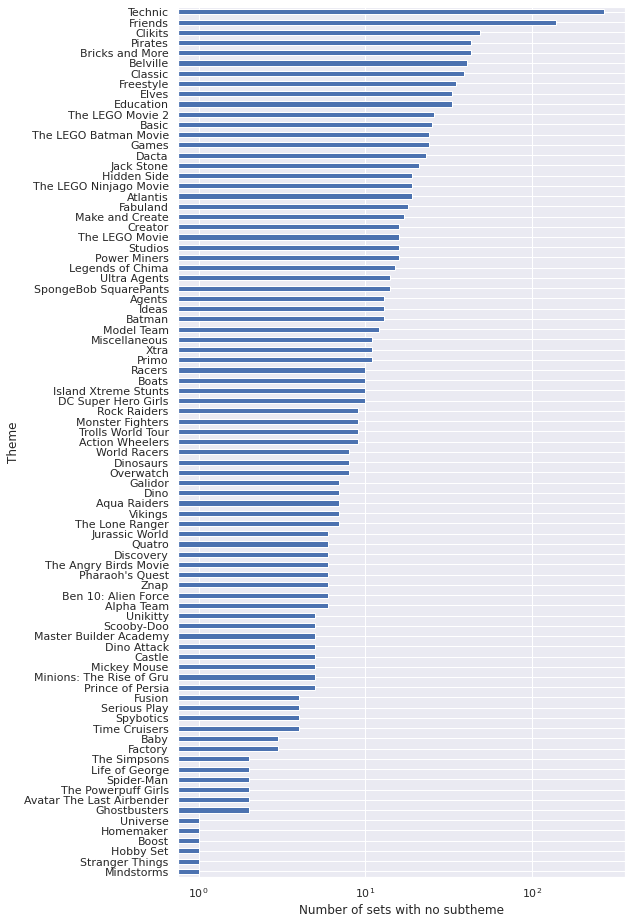When we introduce subtheme-based random effects we will treat these sets with null subtheme values carefully.

Now we check whether or not each subtheme is only ever paired with one theme.

In :
sub_n_themes = (df.groupby("Subtheme")
["Theme"]
.nunique())

In :
ax = (sub_n_themes.value_counts()
.plot.bar(rot=0))

ax.set_xlabel(("Number of themes associated\n"
"with the subtheme"));

ax.set_yscale('log');
ax.set_ylabel("Number of subthemes");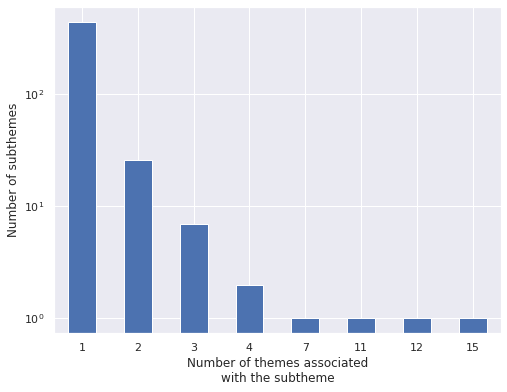In :
(sub_n_themes == 1).mean()

Out:
0.9190871369294605

This plot and calculation show that the vast majority (about 92%) of subthemes are associated with only one theme. We now examine the subthemes that are associated with more than one theme.

In :
ax = (sub_n_themes[sub_n_themes > 1]
.sort_values()
.plot.barh(figsize=(8, 9)))

ax.set_xlabel(("Number of themes associated\n"
"with the subtheme"));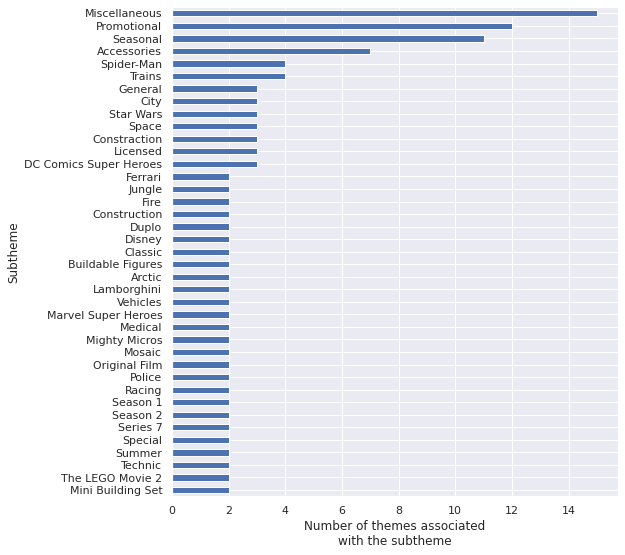The subthemes associated with the most themes make sense, many themes will have "Miscellaneous," "Promotional," "Seasonal," and "Accessories" sets. Of the remaining such subthemes, I personally am curious about the themes that the "Star Wars" subtheme is associated with.

In :
STAR_WARS = "Star Wars"

In :
ax = (df[df["Subtheme"] == STAR_WARS]
["Theme"]
.value_counts()
.plot.barh())

ax.set_xlabel(("Number of sets with\n"
"\"Star Wars\" subtheme"))

ax.invert_yaxis();
ax.set_ylabel("Theme");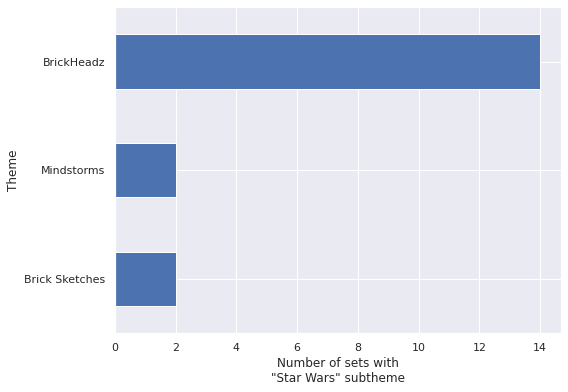These themes make sense, there are "BrickHeadz" of many sorts (in addition to Star Wars, Disney, Harry Potter, and Pets come to mind immediately) and the same reasoning applies to "Brick Sketches" and "Mindstorms."

In order to truly remain faithful to the source data, we will treat theme and subtheme as if they are not nested, even though in reality they are.

## Modeling¶

### Time-varying intercepts, theme-based random effects and slopes¶

We begin by rebuilding the final model from the previous post, which models the relationship between log RRP in 2021 dollars and log piece count using time-varying intercepts and time-constant slopes with theme-based random effects. For full details of this model please consult the previous post.

First we build the feature vector $x$ (standardized log price) and the target vector $y$ (log RRP in 2021 dollars).

In :
pieces = df["Pieces"].values
log_pieces = np.log(df["Pieces"].values)

rrp2021 = df["RRP2021"].values
log_rrp2021 = np.log(rrp2021)

In :
scaler = StandardScaler().fit(log_pieces[:, np.newaxis])

def scale_log_pieces(log_pieces, scaler=scaler):
return scaler.transform(log_pieces[:, np.newaxis])[:, 0]

std_log_pieces = scale_log_pieces(log_pieces)
std_log_pieces_ = shared(std_log_pieces)


We also encode the themes as integer indices and calculate the (standardize) mean log piece count per theme in order to make an adjustment to satisfy the Gauss-Markov criteria.

In :
theme_id, theme_map = df["Theme"].factorize(sort=True)
n_theme = theme_map.size

theme_id_ = shared(theme_id)

In :
theme_mean_std_log_pieces = (pd.Series(std_log_pieces, index=df.index)
.groupby(df["Theme"])
.mean())


Finally we encode the year (in years since 1960) that the set was released for the time-varying component of the intercept.

In :
year = df.index.get_level_values("Year released").year.values
t = year - year.min()
n_year = int(t.max() + 1)

t_ = shared(t)


We now define the final model of the previous post in PyMC3.

In :
def hierarchical_normal(name, shape, μ=None):
if μ is None:
μ = pm.Normal(f"μ_{name}", 0., 2.5)

Δ = pm.Normal(f"Δ_{name}", 0., 1., shape=shape)
σ = pm.HalfNormal(f"σ_{name}", 2.5)

return pm.Deterministic(name, μ + Δ * σ)

def hierarchical_normal_with_mean(name, x_mean, shape,
μ=None, mean_name="γ"):
mean_coef = pm.Normal(f"{mean_name}_{name}", 0., 2.5)

return pm.Deterministic(
name,
hierarchical_normal(f"_{name}", shape, μ=μ) \
+ mean_coef * x_mean
)

In :
def gaussian_random_walk(name, shape, innov_scale=1.):
Δ = pm.Normal(f"Δ_{name}", 0., innov_scale,  shape=shape)

return pm.Deterministic(name, Δ.cumsum())

In :
with pm.Model() as model:
β0_0 = pm.Normal("β0_0", 0., 2.5)

β0_t = gaussian_random_walk("β0_t", n_year, innov_scale=0.1)

β0_theme = hierarchical_normal_with_mean(
"β0_theme",
at.constant(theme_mean_std_log_pieces),
n_theme, μ=0.
)
β0_i = β0_0 + β0_t[t_] + β0_theme[theme_id_]

β_pieces_0 = pm.Normal("β_pieces_0", 0., 2.5)

β_pieces_theme = hierarchical_normal_with_mean(
"β_pieces_theme",
at.constant(theme_mean_std_log_pieces),
n_theme, μ=0.
)

β_pieces = β_pieces_0 + β_pieces_theme

σ = pm.HalfNormal("σ", 5.)
μ = β0_i + β_pieces_theme[theme_id_] * std_log_pieces_ - 0.5 * σ**2

obs = pm.Normal("obs", μ, σ, observed=log_rrp2021)


We are finally ready to sample from the posterior distribution of this model given the Brickset data.

In :
CHAINS = 3
SEED = 123456789

SAMPLE_KWARGS = {
'cores': CHAINS,
'random_seed': [SEED + i for i in range(CHAINS)],
'return_inferencedata': True
}

In :
with model:
trace = pm.sample(**SAMPLE_KWARGS)

Auto-assigning NUTS sampler...
Multiprocess sampling (3 chains in 3 jobs)
NUTS: [β0_0, Δ_β0_t, γ_β0_theme, Δ__β0_theme, σ__β0_theme, β_pieces_0, γ_β_pieces_theme, Δ__β_pieces_theme, σ__β_pieces_theme, σ]

100.00% [6000/6000 09:51<00:00 Sampling 3 chains, 0 divergences]
Sampling 3 chains for 1_000 tune and 1_000 draw iterations (3_000 + 3_000 draws total) took 592 seconds.
The estimated number of effective samples is smaller than 200 for some parameters.


The standard sampling diagnostics show no cause for concern.

In :
def plot_diagnostics(trace, axes=None, min_mult=0.995, max_mult=1.005):
if axes is None:
fig, axes = plt.subplots(ncols=2, sharex=False, sharey=False,
figsize=(16, 6))

az.plot_energy(trace, ax=axes)

rhat = az.rhat(trace).max()
axes.barh(np.arange(len(rhat.variables)), rhat.to_array(),
tick_label=list(rhat.variables.keys()))
axes.axvline(1, c='k', ls='--')

axes.set_xlim(
min_mult * min(rhat.min().to_array().min(), 1),
max_mult * max(rhat.max().to_array().max(), 1)
)
axes.set_xlabel(r"$\hat{R}$")

axes.set_ylabel("Variable")

return fig, axes

In :
plot_diagnostics(trace);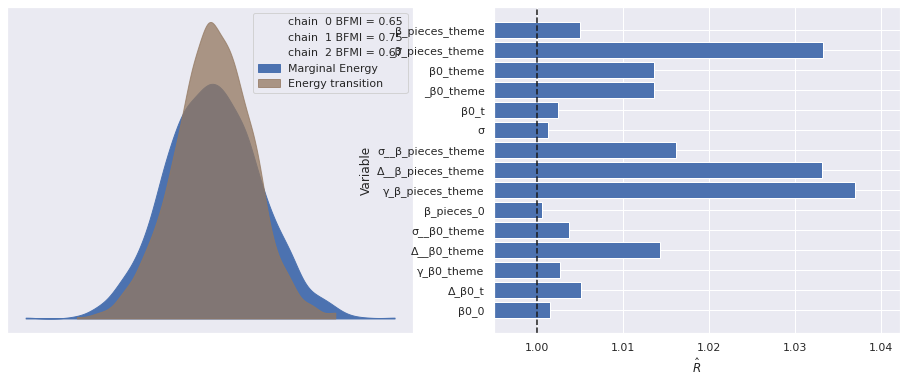We now sample from the posterior predictive distribution of the model and visualize the resulting residuals.

In :
with model:
pp_data = pm.sample_posterior_predictive(trace)

df["pred"] = pp_data["obs"].mean(axis=0)
df["resid"] = log_rrp2021 - df["pred"]

100.00% [3000/3000 00:01<00:00]
In :
ax = sns.scatterplot(x="Pieces", y="resid",
data=df.reset_index(),
color='C0', alpha=0.1);
(df.groupby(df["Pieces"].round(-2))
["resid"]
.mean()
.plot(c='k', label="Bucketed average",
ax=ax));

ax.set_xscale('log');

ax.set_ylim(-2, 2);
ax.set_ylabel("Log RRP residual (2021 $)"); ax.legend(loc='upper left');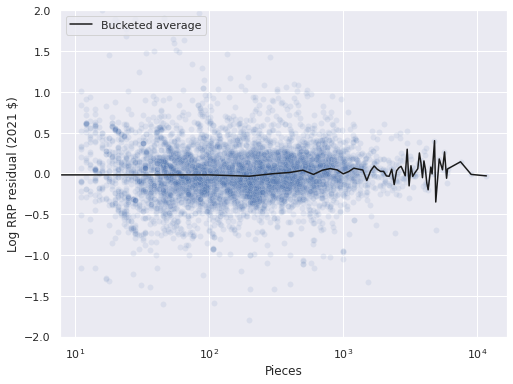In : ax = (df.groupby(level="Year released") ["resid"] .mean() .plot(c='k', label="Year average")); strip_ax = ax.twiny() sns.stripplot(x="Year released", y="resid", data=df.reset_index(), jitter=1.5, color='C0', alpha=0.1, ax=strip_ax); strip_ax.set_axis_off(); ax.set_ylim(-2, 2); ax.set_ylabel("Log RRP residual (2021$)");

ax.legend(loc='upper left');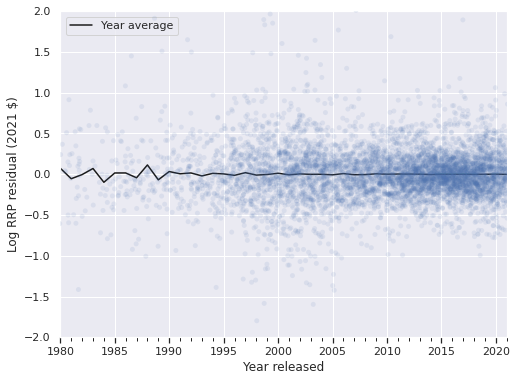The residuals are reasonably well-centered around zero when grouped by piece and year as expected from the previous post.

### Adding subtheme-based random effects and slopes¶

We now encode subthemes as integer identifiers.

In :
sub_id, sub_map = df["Subtheme"].factorize(sort=True)
n_sub = sub_map.size

sub_id_ = shared(sub_id)


Note that factorize encodes null values as -1 by default, so we see that the number of such entries in sub_id matches the number of sets with null subtheme.

In :
(sub_id == -1).sum()

Out:
1416
In :
(df["Subtheme"]
.isnull()
.sum())

Out:
1416

We build a mask indicating which subthemes are null.

In :
sub_isnull = sub_id == -1
sub_isnull_ = shared(sub_isnull)


We also calculate the average (standardized) log number of pieces for each set in a subtheme.

In :
sub_mean_std_log_pieces = (pd.Series(std_log_pieces, index=df.index)
.groupby(df["Subtheme"])
.mean())


Recall from the previous post that $j(i)$ represents the index of the theme of the $i$-th set. In similar fashion, let $k(i)$ denote the index of the subtheme of the $i$-th set.

Our model with additional random effects and slopes for subthemes is as follows.

The expected value of the slope is $\beta_0^0 \sim N(0, 2.5^2)$.

In :
with pm.Model() as sub_model:
β0_0 = pm.Normal("β0_0", 0., 2.5)


The time-varying component of the intercept is

$$\beta_{0, t} \sim \text{GaussianRandomWalk}\left(0, (0.1)^2\right).$$
In :
with sub_model:
β0_t = gaussian_random_walk("β0_t", n_year, innov_scale=0.1)

\begin{align*} \gamma_0 & \sim N(0, 2.5^2) \\ \sigma_0^{\text{theme}} & \sim \textrm{HalfNormal}(2.5^2) \\ \beta_{0, j}^{\text{theme}} & \sim N\left(\gamma_0 \cdot \bar{\tilde{x}}_j, \left(\sigma_0^{\text{theme}}\right)^2\right). \end{align*}

Recall that here $\tilde{x}_i$ is the standardized log piece count of the $i$-th set and $\bar{\tilde{x}}_j$ is the average standardized log piece count of all sets in the $j$-th theme.

In :
with sub_model:
β0_theme = hierarchical_normal_with_mean(
"β0_theme",
at.constant(theme_mean_std_log_pieces),
n_theme, μ=0.
)


We now include the sub-theme based random intercepts. All sets with null subtheme are given the same subtheme effect, $\beta_{0, -1}^{\text{sub}}$. We use

\begin{align*} \beta_{0, -1}^{\text{sub}}, \lambda_0^{\text{pieces}} & \sim N(0, 2.5^2) \\ \sigma_0^{\text{sub}} & \sim \text{HalfNormal}(2.5^2) \\ \beta_{0, k}^{\text{sub}} & \sim N\left(\lambda_0^{\text{pieces}} \cdot \check{\tilde{x}}_k, \left(\sigma_0^{\text{sub}}\right)^2\right). \end{align*}

Here $\check{\tilde{x}}_k$ is the average standardized log piece count of all sets in the $k$-th subtheme.

In :
with sub_model:
β0_sub_null = pm.Normal("β0_sub_null", 0., 2.5)
β0_sub_nn = hierarchical_normal_with_mean(
"β0_sub_nn",
at.constant(sub_mean_std_log_pieces),
n_sub, μ=0., mean_name="λ"
)
β0_sub_i = at.switch(
sub_isnull_,
β0_sub_null,
β0_sub_nn[at.clip(sub_id_, 0, n_sub)]
)


Assembling the intercept terms, we get

$$\beta_{0, i} = \beta_{0, 0} + \beta_{0, t(i)} + \beta_{0, j(i)}^{\text{theme}} + \beta_{0, k(i)}^{\text{sub}}.$$
In :
with sub_model:
β0_i = β0_0 + β0_t[t_] + β0_theme[theme_id_] + β0_sub_i


The varying slopes, $\beta_{\text{pieces}, i}$, are defined similarly, just without a time-varying component.

In :
with sub_model:
β_pieces_0 = pm.Normal("β_pieces_0", 0., 2.5)

β_pieces_theme = hierarchical_normal_with_mean(
"β_pieces_theme",
at.constant(theme_mean_std_log_pieces),
n_theme, μ=0.
)

β_pieces_sub_null = pm.Normal("β_pieces_sub_null", 0., 2.5)
β_pieces_sub_nn = hierarchical_normal_with_mean(
"β_pieces_sub_nn",
at.constant(sub_mean_std_log_pieces),
n_sub, μ=0., mean_name="λ"
)
β_pieces_sub_i = at.switch(
sub_isnull_,
β_pieces_sub_null,
β_pieces_sub_nn[at.clip(sub_id_, 0, n_sub)]
)

β_pieces_i = β_pieces_0 + β_pieces_theme[theme_id_] + β_pieces_sub_i


Finally, we specify the likelihood of this model and sample from its posterior distribution.

In :
with sub_model:
σ = pm.HalfNormal("σ", 5.)
μ = β0_i + β_pieces_i * std_log_pieces_ - 0.5 * σ**2

obs = pm.Normal("obs", μ, σ, observed=log_rrp2021)

In :
with sub_model:
sub_trace = pm.sample(**SAMPLE_KWARGS)

Auto-assigning NUTS sampler...
Multiprocess sampling (3 chains in 3 jobs)
NUTS: [β0_0, Δ_β0_t, γ_β0_theme, Δ__β0_theme, σ__β0_theme, β0_sub_null, λ_β0_sub_nn, Δ__β0_sub_nn, σ__β0_sub_nn, β_pieces_0, γ_β_pieces_theme, Δ__β_pieces_theme, σ__β_pieces_theme, β_pieces_sub_null, λ_β_pieces_sub_nn, Δ__β_pieces_sub_nn, σ__β_pieces_sub_nn, σ]

100.00% [6000/6000 17:10<00:00 Sampling 3 chains, 0 divergences]
Sampling 3 chains for 1_000 tune and 1_000 draw iterations (3_000 + 3_000 draws total) took 1031 seconds.
The number of effective samples is smaller than 25% for some parameters.


The sampling diagnostics show no cause for concern.

In :
plot_diagnostics(sub_trace);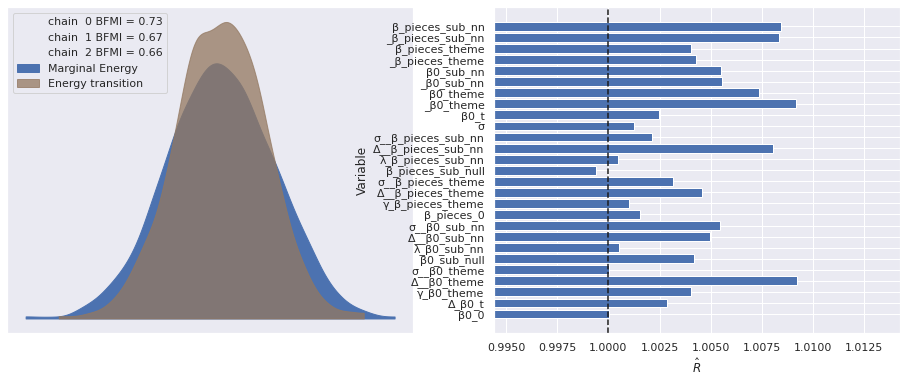We now sample from the posterior predictive distribution of the model and compare the resulting residuals to those of the baseline model.

In :
with sub_model:
pp_sub_data = pm.sample_posterior_predictive(sub_trace)

df["sub_pred"] = pp_sub_data["obs"].mean(axis=0)
df["sub_resid"] = log_rrp2021 - df["sub_pred"]

100.00% [3000/3000 00:02<00:00]
In :
fig, (ax, sub_ax) = plt.subplots(
ncols=2, sharex=True, sharey=True,
figsize=(2 * FIGSIZE, FIGSIZE)
)

sns.scatterplot(x="Pieces", y="resid",
data=df.reset_index(),
color='C0', alpha=0.1,
ax=ax);
(df.groupby(df["Pieces"].round(-2))
["resid"]
.mean()
.plot(c='k', label="Bucketed average",
ax=ax));

ax.set_xscale('log');

ax.set_ylim(-2, 2);
ax.set_ylabel("Log RRP residual (2021 $)"); ax.legend(loc='upper left'); ax.set_title("Baseline model"); sns.scatterplot(x="Pieces", y="sub_resid", data=df.reset_index(), color='C0', alpha=0.1, ax=sub_ax); (df.groupby(df["Pieces"].round(-2)) ["sub_resid"] .mean() .plot(c='k', ax=sub_ax)); sub_ax.set_title("Subtheme model"); fig.tight_layout();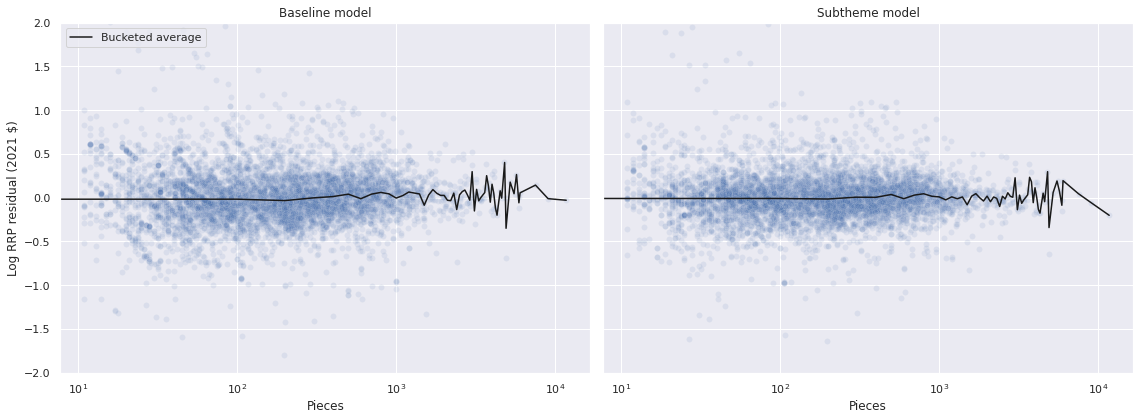In : fig, (ax, sub_ax) = plt.subplots( ncols=2, sharex=True, sharey=True, figsize=(2 * FIGSIZE, FIGSIZE) ) (df.groupby(level="Year released") ["resid"] .mean() .plot(c='k', label="Year average", ax=ax)); strip_ax = ax.twiny() sns.stripplot(x="Year released", y="resid", data=df.reset_index(), jitter=1.5, color='C0', alpha=0.1, ax=strip_ax); strip_ax.set_axis_off(); ax.set_ylim(-2, 2); ax.set_ylabel("Log RRP residual (2021$)");

ax.legend(loc='upper left');
ax.set_title("Baseline model");

(df.groupby(level="Year released")
["sub_resid"]
.mean()
.plot(c='k', label="Year average",
ax=sub_ax));

sub_strip_ax = sub_ax.twiny()
sns.stripplot(x="Year released", y="sub_resid",
data=df.reset_index(),
jitter=1.5,
color='C0', alpha=0.1,
ax=sub_strip_ax);

sub_strip_ax.set_axis_off();

sub_ax.set_title("Subtheme model");

fig.tight_layout();